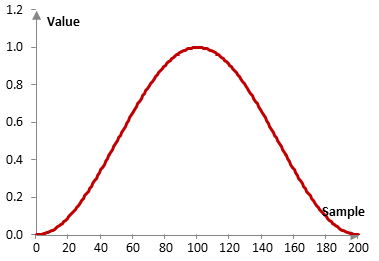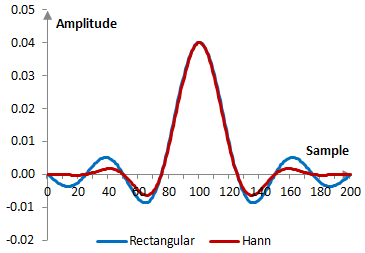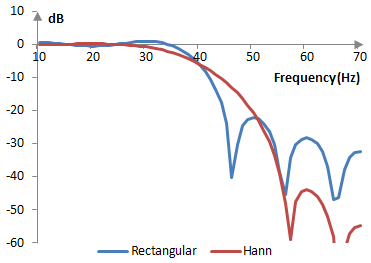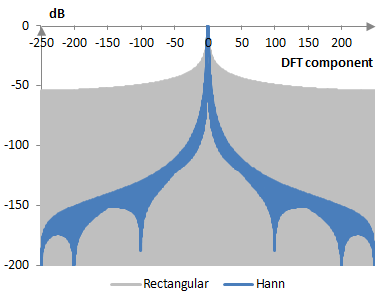# Hann window

The Hann window coefficients are given by the following formula

$$a(k)=0.5 * (1-\cos(\frac{2\pi\,k}{N-1}))$$

where N is the length of the filter and k = 0, 1, …, N – 1.

The Hann window belongs to the family of Hamming windows. The derivation of the Hann window is shown in the topic Hamming window. The Hann window is also a poiwer of cosine window (α = 2). When the Hann window is multiplied by the Poisson window, the result is the Hann-Poisson window.

## An example Hann window

Consider a finite impulse response (FIR) low pass filter of length N = 201. The following is the Hann window.Given a sampling frequency of 2000 Hz and a filter cutoff frequency of 40 Hz, the impulse response of the filter with a rectangular window (with no window) and with the Hann window is as follows.The magnitude response of the same filter is shown on the graph below.## Measures for the Hann window

The following is a comparison of the discrete Fourier transform of the Hann window and the rectangular window.The Hann window measures are as follows.

 Coherent gain 0.50 Equivalent noise bandwidth 1.50 Processing gain -1.77 dB Scalloping loss -1.42 dB Worst case processing loss -3.19 dB Highest sidelobe level -31.5 dB Sidelobe falloff -20.7 dB / octave, -68.9 dB / decade Main lobe is -3 dB 1.44 bins Main lobe is -6 dB 2.00 bins Overlap correlation at 50% overlap 0.165 Amplitude flatness at 50% overlap 1.000 Overlap correlation at 75% overlap 0.658 Amplitude flatness at 75% overlap 1.000

Window

### Filtered HTML

• Freelinking helps you easily create HTML links. Links take the form of [[indicator:target|Title]]. By default (no indicator): Click to view a local node.